150416 Epaper

 A Section B SectionC Section D Section150415 Epaper

 A Section B Section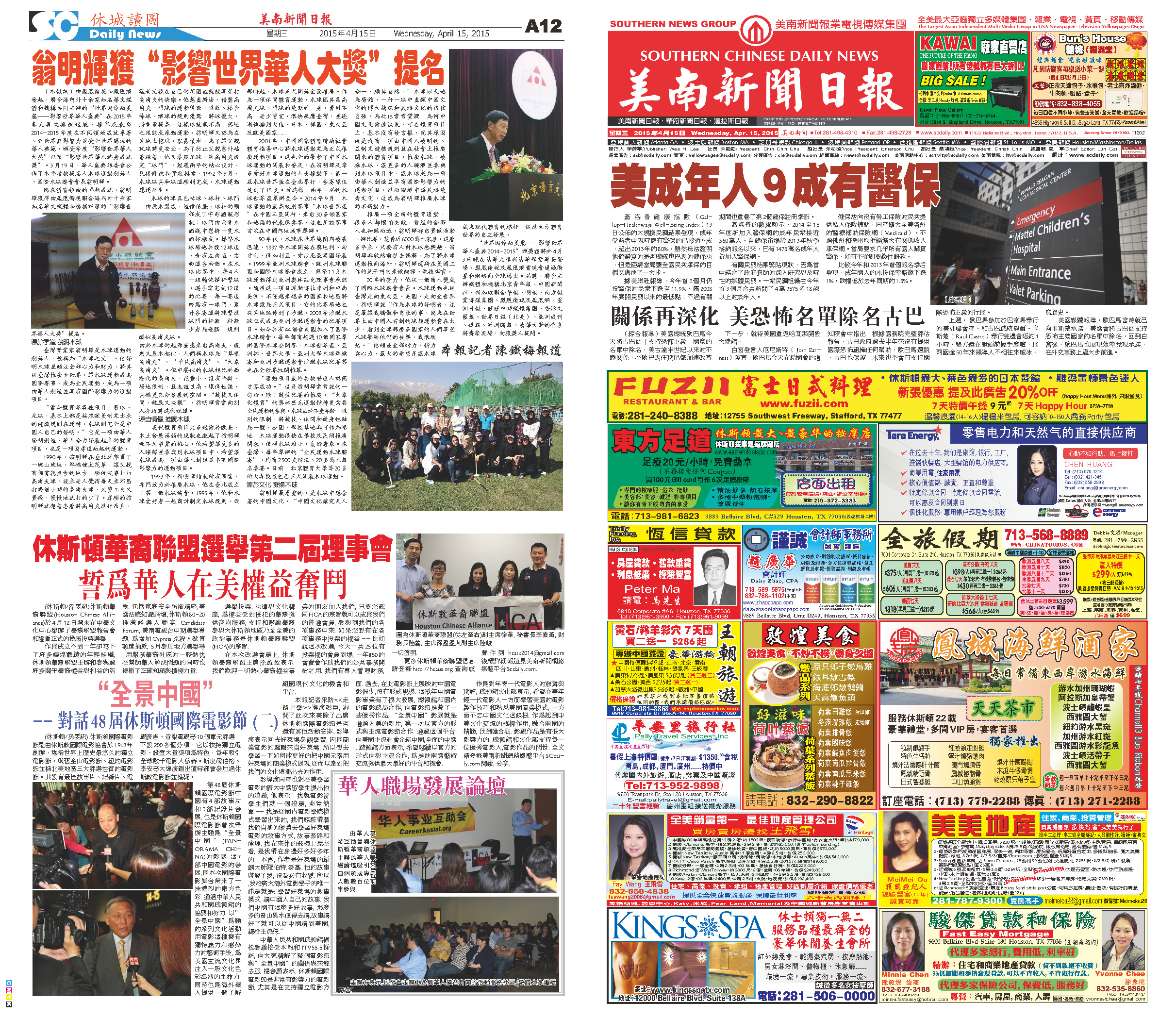C Section D Section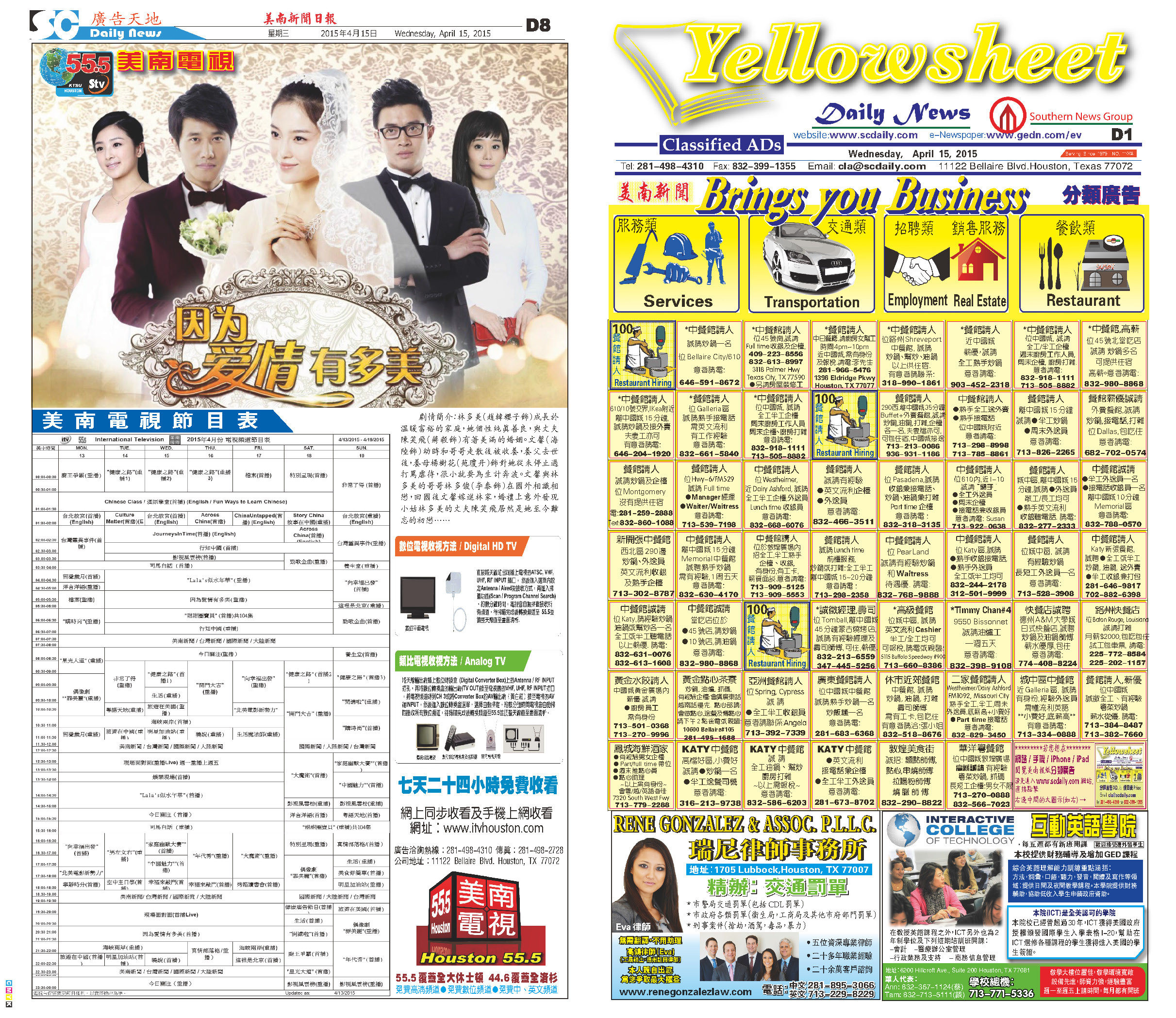150413 Epaper

 A Section B Section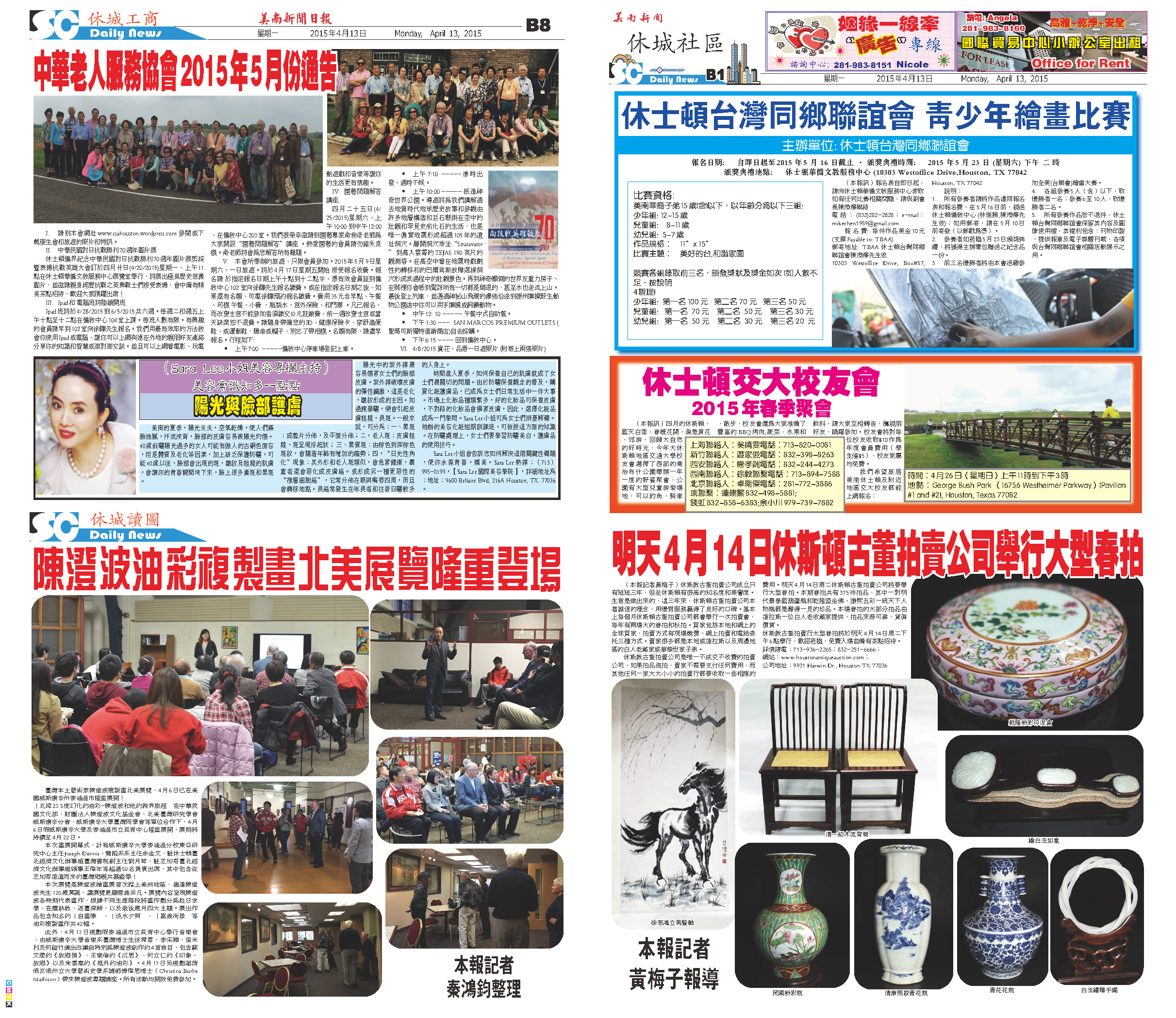C Section D Section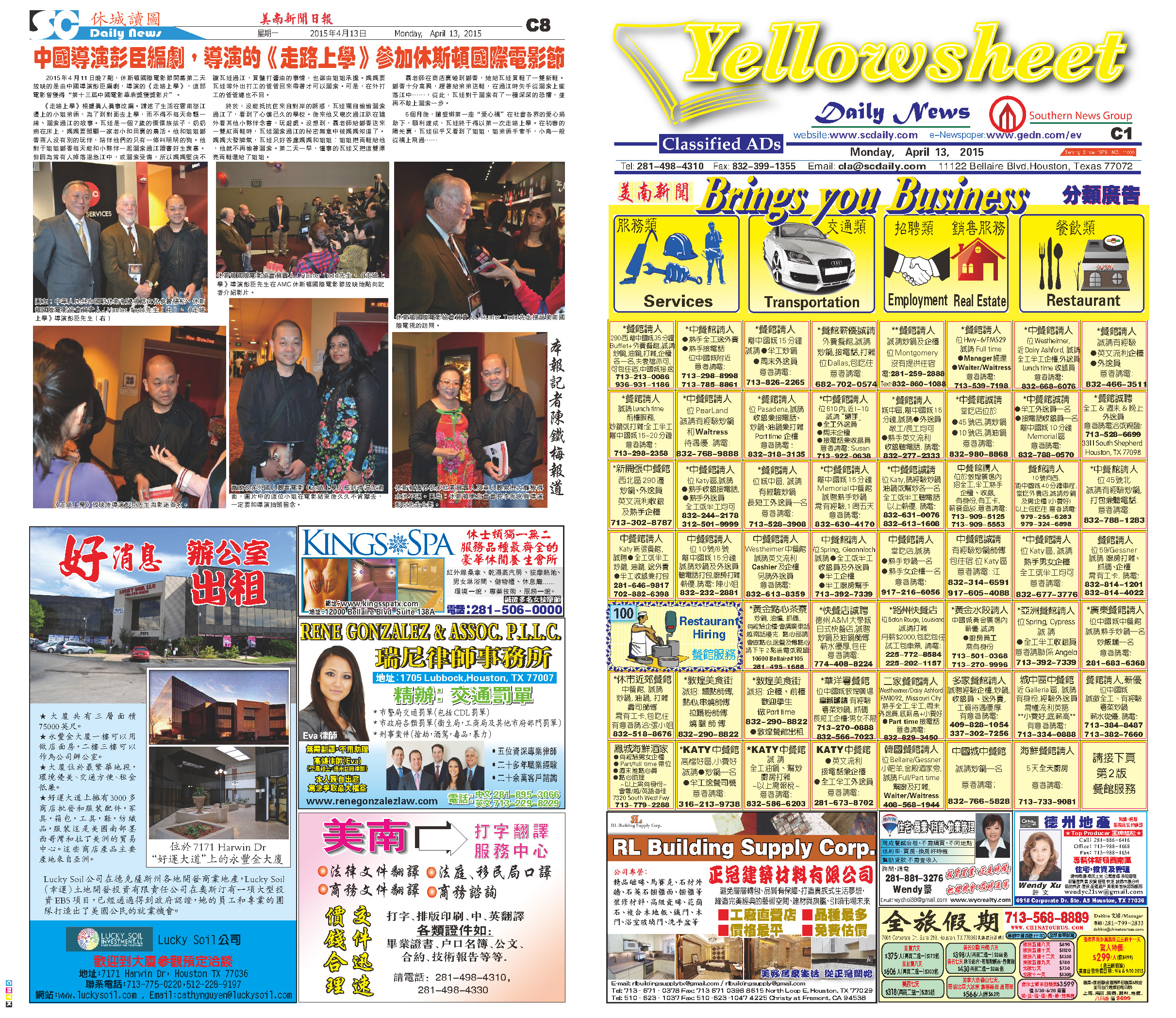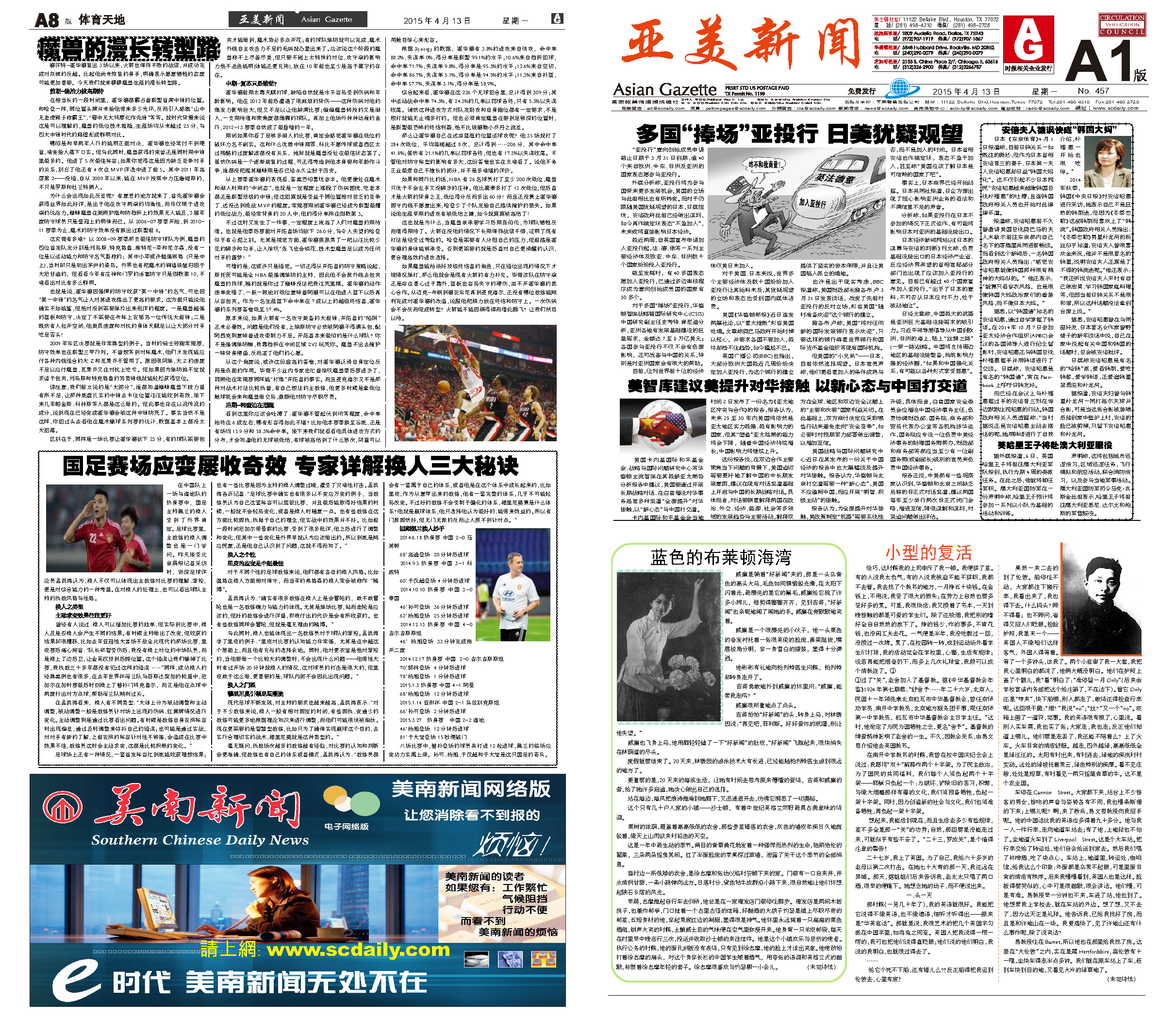150412 Epaper

 A Section B SectionC Section D Section150411 Epaper

 A Section B SectionC Section D Section150410 Epaper

 A Section B SectionC Section D Section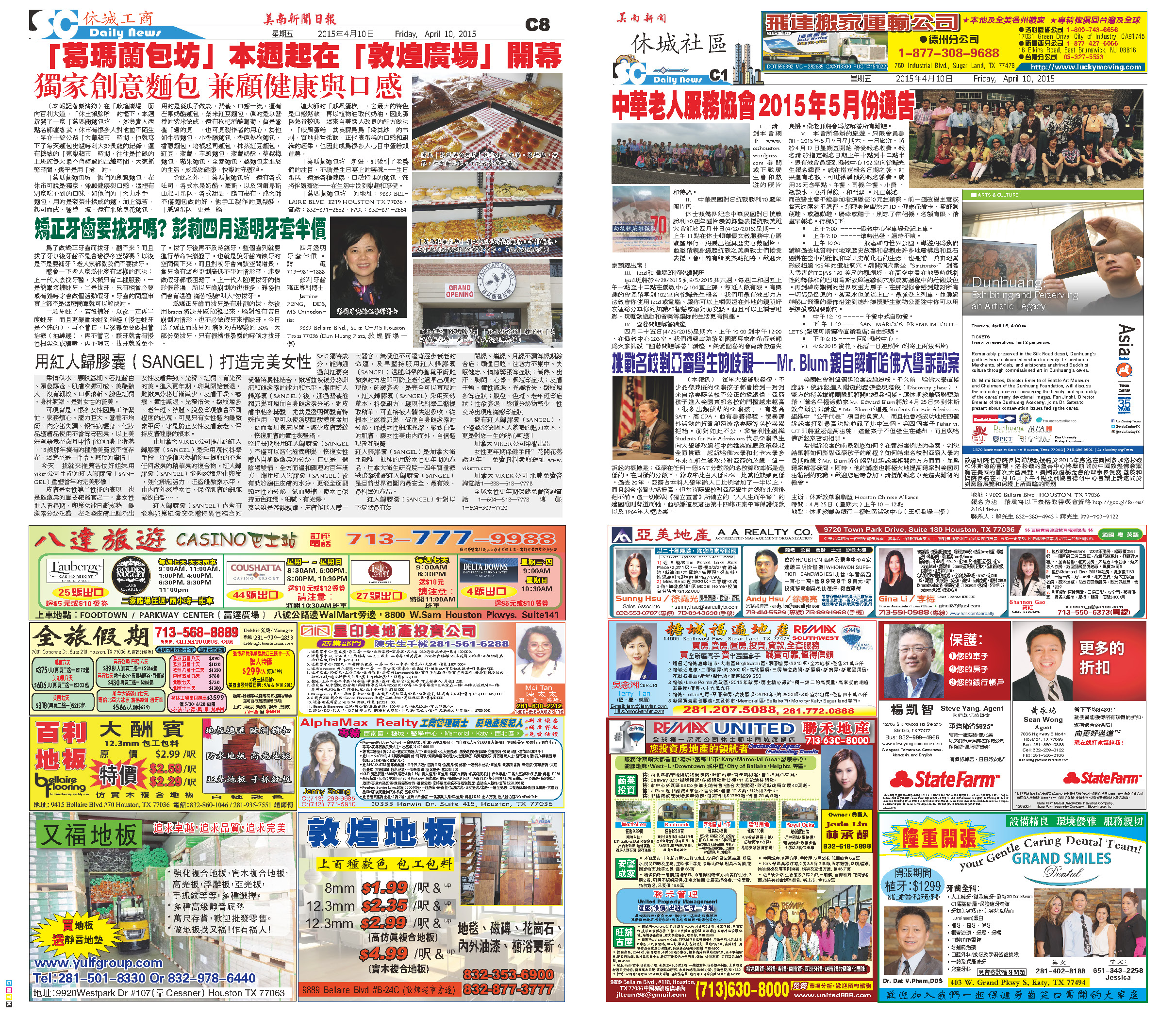150409 Epaper

 A Section B SectionC Section D Section150408 Epaper

 A Section B SectionC Section D Section150406 Epaper

 A Section B SectionC Section D Section150407 Epaper

 A Section B SectionC Section D Section150405 Epaper

 A Section B SectionC Section D Section